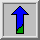# Distance Metrics

It is often useful in image processing to be able to calculate the distance between two pixels in an image, but this is not as straightforward as it seems. The presence of the pixel grid makes several so-called distance metrics possible which often give different answers to each other for the distance between the same pair of points. We consider the three most important ones.

## Euclidean Distance

This is the familiar straight line distance that most people are familiar with. If the two pixels that we are considering have coordinates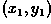and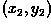, then the Euclidean distance is given by: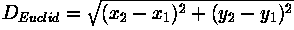## City Block Distance

Also known as the Manhattan distance. This metric assumes that in going from one pixel to the other it is only possible to travel directly along pixel grid lines. Diagonal moves are not allowed. Therefore the `city block' distance is given by: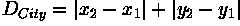## Chessboard Distance

This metric assumes that you can make moves on the pixel grid as if you were a King making moves in chess, i.e. a diagonal move counts the same as a horizontal move. This means that the metric is given by: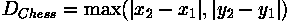Note that the last two metrics are usually much faster to compute than the Euclidean metric and so are sometimes used where speed is critical but accuracy is not too important.